Get instant live expert help with Excel or Google Sheets“My Excelchat expert helped me in less than 20 minutes, saving me what would have been 5 hours of work!”

#### Post your problem and you'll get expert help in seconds

Your message must be at least 40 characters
Our professional experts are available now. Your privacy is guaranteed.

# Sum Bottom N Values with Criteria

Excel allows a user to sum bottom N values with criteria using the SUM, SMALL and IF function. The final formula has to be converted to array function with ctrl+shift+enter in order to work properly. This step by step tutorial will assist all levels of Excel users to sum the smallest N values from the array based on the defined criteria.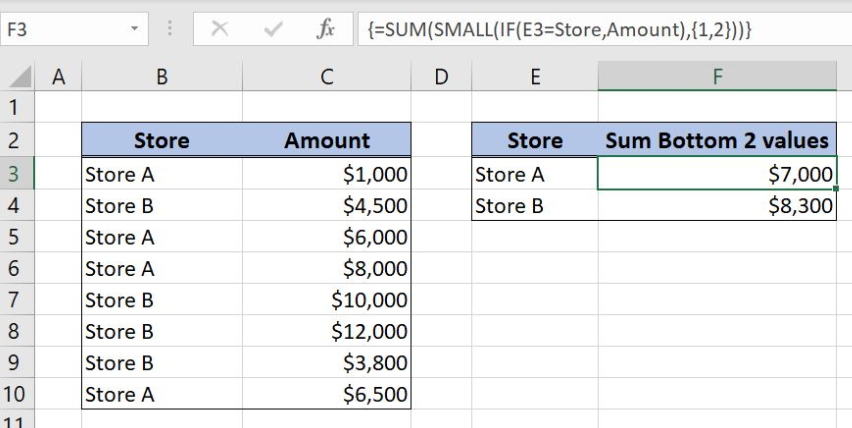Figure 1. Sum bottom 2 amounts for each store

## Syntax of the SUM Formula

`=SUM(number1, [number2], ...)`

The parameters of the SUM function are:

• number1, [number2] – numbers to sum

## Syntax of the SMALL Formula

`=SMALL(array, k)`

The parameters of the SUM function are:

• array– cell range where we want to find the k-th smallest value
• k– position of the value in the array starting from the smallest value

## Syntax of the IF Formula

The generic formula for the IF function is:

`=IF(logical_test, value_if_true, value_if_false)`

The parameters of the IF function are:

• logical_test – a logical expression that we want to check
• value_if_true – a value which the function returns if a logical_test is TRUE
• value_if_false – a value which the function returns if a logical_test is FALSE.

## Setting up Our Data to Sum bottom n values with criteria

Our first table consists of 2 columns: “Store” (column B) and “Amount” (column C). The second one has 2 columns: “Store” (Column E) and “Sum Bottom 2 values” (Column F). The idea is to sum the smallest two values from column “Amount” per each store.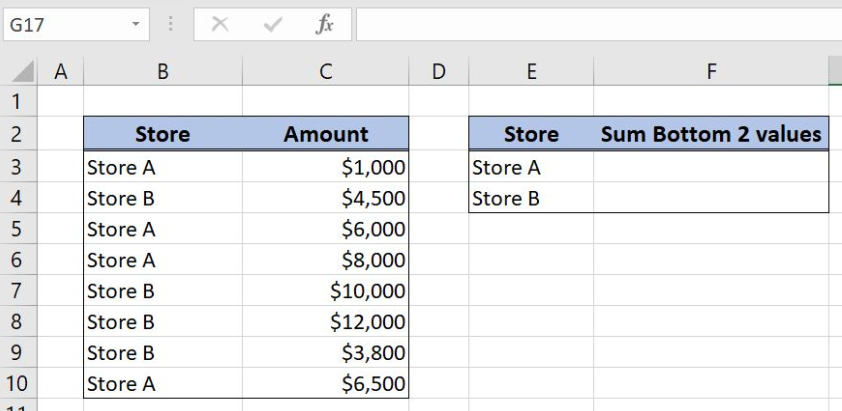Figure 2. Table structure for summing the smallest two values

## Sum with Criteria Bottom Two Values with SUM, SMALL and IF Functions

We want to sum the smallest two values from column “Amount”. Criteria for summing is each store in column E, Store A, and Store B. In order to make the formula more clear, we will create a named range Store for cell range B3:B10 and a named range Amount for the cell range C3:C10.

To create a named range we should follow the steps:

• Select the cell range that should be named
• Click on the name box in Excel
• Write the name for the cell range and press enter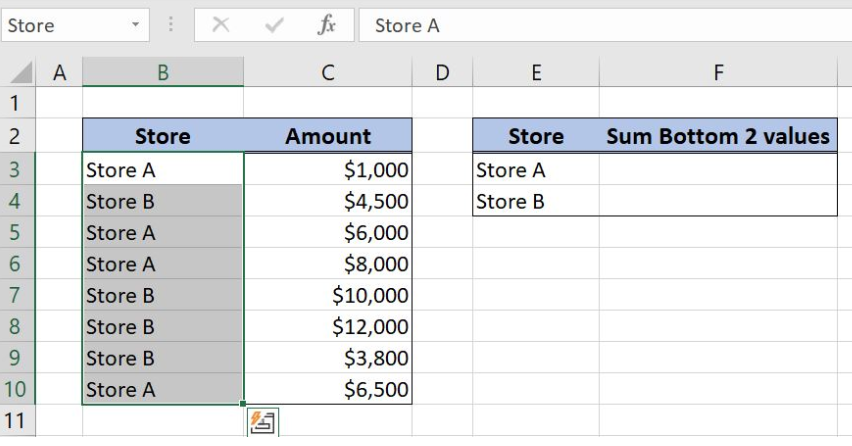Figure 3. Creating a named range Store for column “Store”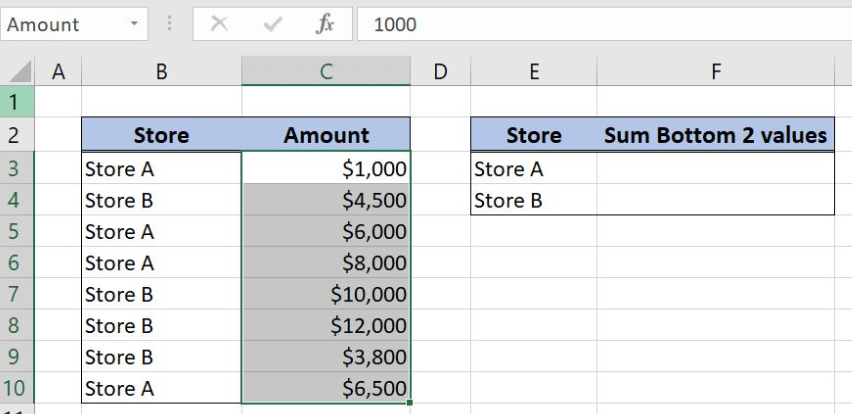Figure 4. Creating a named range Amount for column “Amount”

The formula for summing bottom two values with criteria looks like:

`{=SUM(SMALL(IF(E3=Store,Amount),{1,2}))}`

The number1 in the SUM function is the formula part SMALL(IF(E3=Store,Amount),{1,2})). In SMALL function array is formula part IF(E3=Store,Amount) while the k is the array {1,2}. The logical_test in IF function is checking if the store in the cell E3 is equal to the named range Store, E3=Store. The value_if_true argument is the named range Amount.

To apply the formula we need to follow these steps:

• Select cell F3 and click on it
• Insert the formula: `=SUM(SMALL(IF(E3=Store,Amount),{1,2}))`
• Press ctrl, shift and enter simultaneously to convert the formula in the array function
• Drag the formula down to the other cells in the column by clicking and dragging the little “+” icon at the bottom-right of the cell.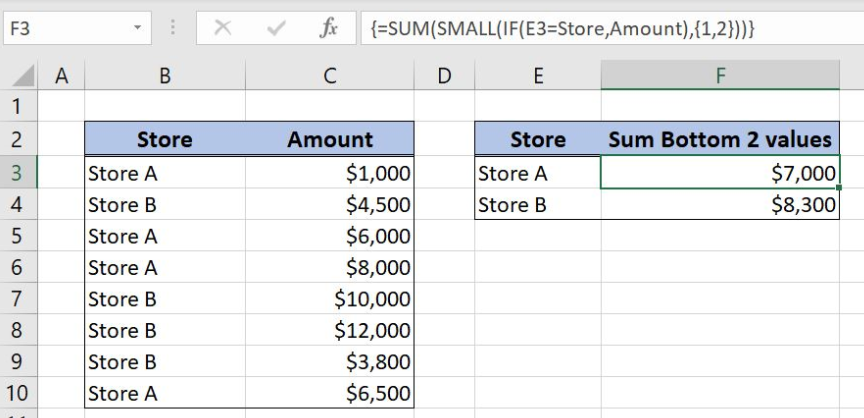Figure 5. Sum the smallest two values from column “Amount” per each store

The SMALL function returns the 1st and the 2nd smallest values from the array because k argument is defined as an array {1,2}. Array argument in the SMALL function depends on the criteria (Store A or Store B) and this is why we are using the IF function, IF(E3=Store,Amount).

As a result, only values from the Store A will go into the array in SMALL function:

`SMALL({1000;FALSE;6000;8000;FALSE;FALSE;FALSE;6500},{1,2})`

SUM function ignores FALSE values and summarizes the smallest two values: {1000,6000}. Formula result is number 7000, the sum of the smallest 2 values in column “Amount” for the Store A.

Most of the time, the problem you will need to solve will be more complex than a simple application of a formula or function. If you want to save hours of research and frustration, try our live Excelchat service! Our Excel Experts are available 24/7 to answer any Excel question you may have. We guarantee a connection within 30 seconds and a customized solution within 20 minutes.

### Did this post not answer your question? Get a solution from connecting with the expert.Another blog reader asked this question today on Excelchat:## Subscribe to Excelchat.coAnother blog reader asked this question today on Excelchat: